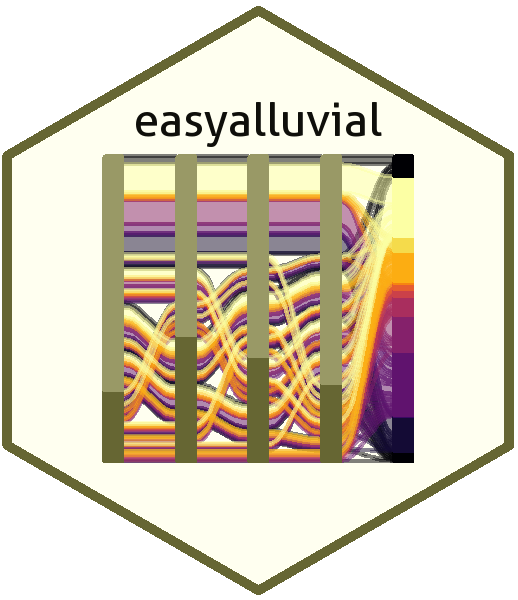easyalluvialAlluvial plots are similar to sankey diagrams and visualise categorical data over multiple dimensions as flows. Rosval et. al. 2010 Their graphical grammar however is a bit more complex then that of a regular x/y plots. The `ggalluvial` package made a great job of translating that grammar into `ggplot2` syntax and gives you many option to tweak the appearance of an alluvial plot, however there still remains a multi-layered complexity that makes it difficult to use ‘ggalluvial’ for explorative data analysis. ‘easyalluvial’ provides a simple interface to this package that allows you to produce a decent alluvial plot from any dataframe in either long or wide format from a single line of code while also handling continuous data. It is meant to allow a quick visualisation of entire dataframes with a focus on different colouring options that can make alluvial plots a great tool for data exploration.

Features

• plot alluvial graph with a single line of code of a given dataframe
• support for wide and long data format (wiki, wide vs. long/narrow data)
• automatically transforms numerical to categorical data
• helper functions for variable selection
• convenient parameters for coloring and ordering
• marginal histograms
• model agnostic partial dependence and model response alluvial plots with 4 dimensions

Installation

CRAN

``install.packages('easyalluvial')``

Development Version

``````
# install.packages("devtools")
devtools::install_github("erblast/easyalluvial")``````

Tutorials

In order to learn about all the features an how they can be useful check out the following tutorials:

Examples

``````suppressPackageStartupMessages( require(tidyverse) )
suppressPackageStartupMessages( require(easyalluvial) )``````

Alluvial from data in wide format

Sample Data

``````
mpg cyl disp hp drat wt qsec vs am gear carb ids
21.0 6 160 110 3.90 2.620 16.46 V manual 4 4 Mazda RX4
21.0 6 160 110 3.90 2.875 17.02 V manual 4 4 Mazda RX4 Wag
22.8 4 108 93 3.85 2.320 18.61 S manual 4 1 Datsun 710
21.4 6 258 110 3.08 3.215 19.44 S automatic 3 1 Hornet 4 Drive
18.7 8 360 175 3.15 3.440 17.02 V automatic 3 2 Hornet Sportabout
18.1 6 225 105 2.76 3.460 20.22 S automatic 3 1 Valiant

Plot

Continuous Variables will be automatically binned as follows.

• High, High (HH)
• Medium, High (MH)
• Medium (M)
• Medium, Low (ML)
• Low, Low (LL)
``````
alluvial_wide( data = mtcars2
, max_variables = 5
, fill_by = 'first_variable' )``````Alluvial from data in long format

Sample Data

``knitr::kable( head(quarterly_flights) )``
tailnum carrier origin dest qu mean_arr_delay
N0EGMQ LGA BNA MQ MQ LGA BNA Q1 on_time
N0EGMQ LGA BNA MQ MQ LGA BNA Q2 on_time
N0EGMQ LGA BNA MQ MQ LGA BNA Q3 on_time
N0EGMQ LGA BNA MQ MQ LGA BNA Q4 on_time
N11150 EWR MCI EV EV EWR MCI Q1 late
N11150 EWR MCI EV EV EWR MCI Q2 late

Plot

``````
alluvial_long( quarterly_flights
, key = qu
, value = mean_arr_delay
, id = tailnum
, fill = carrier )``````Marginal Histograms

``````alluvial_wide( data = mtcars2
, max_variables = 5
, fill_by = 'first_variable' ) %>%Partial Dependence Alluvial Plots

Alluvial plots are capable of displaying higher dimensional data on a plane, thus lend themselves to plot the response of a statistical model to changes in the input data across multiple dimensions. The practical limit here is 4 dimensions while conventional partial dependence plots are limited to 2 dimensions.

Briefly the 4 variables with the highest feature importance for a given model are selected and 5 values spread over the variable range are selected for each. Then a grid of all possible combinations is created. All none-plotted variables are set to the values found in the first row of the training data set. Using this artificial data space model predictions are being generated. This process is then repeated for each row in the training data set and the overall model response is averaged in the end. Each of the possible combinations is plotted as a flow which is coloured by the bin corresponding to the average model response generated by that particular combination.

``````
df = select(mtcars2, -ids)
m = randomForest::randomForest( disp ~ ., df)
imp = m\$importance

dspace = get_data_space(df, imp, degree = 4)

pred = get_pdp_predictions(df, imp
, m
, degree = 4
, bins = 5)

p = alluvial_model_response(pred, dspace, imp
, degree = 4, method = 'pdp'
, stratum_label_size = 2.75)

p_grid = add_marginal_histograms(p, df, plot = F) %>%